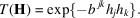International
Tables for
Crystallography
Volume B
Reciprocal space
Edited by U. Shmueli

International Tables for Crystallography (2006). Vol. B, ch. 1.2, pp. 18-19   | 1 | 2 |

Section 1.2.10. The vibrational probability distribution and its Fourier transform in the harmonic approximation

P. Coppensa*

aDepartment of Chemistry, Natural Sciences & Mathematics Complex, State University of New York at Buffalo, Buffalo, New York 14260-3000, USA
Correspondence e-mail: coppens@acsu.buffalo.edu

1.2.10. The vibrational probability distribution and its Fourier transform in the harmonic approximation

| top | pdf |

For a harmonic oscillator, the probability distribution averaged over all populated energy levels is a Gaussian, centred at the equilibrium position. For the three-dimensional isotropic harmonic oscillator, the distribution is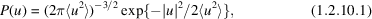where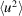is the mean-square displacement in any direction.

The corresponding trivariate normal distribution to be used for anisotropic harmonic motion is, in tensor notation,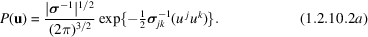Here σ is the variance–covariance matrix, with covariant components, and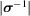is the determinant of the inverse of σ. Summation over repeated indices has been assumed. The corresponding equation in matrix notation is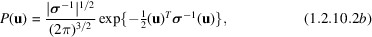where the superscript T indicates the transpose.

The characteristic function, or Fourier transform, of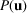is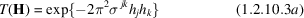or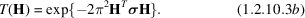With the change of variable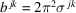, (1.2.10.3a)becomes